Anúncio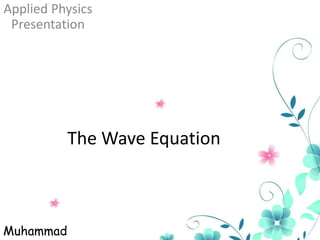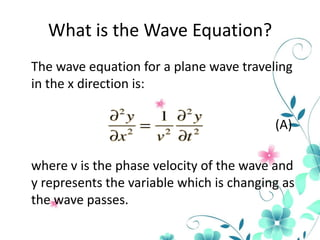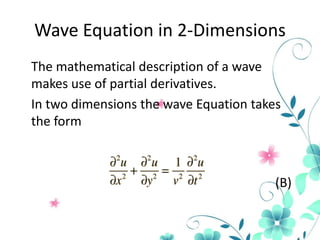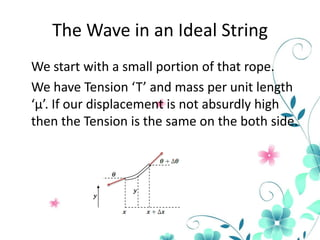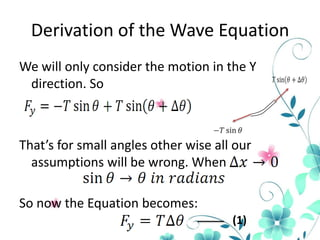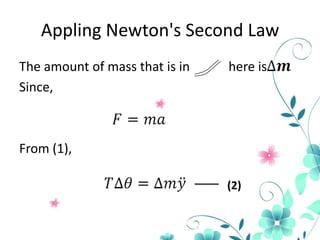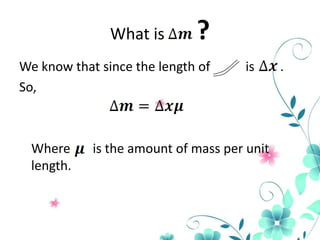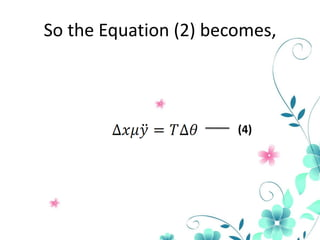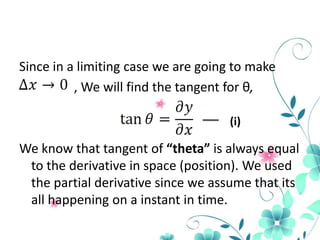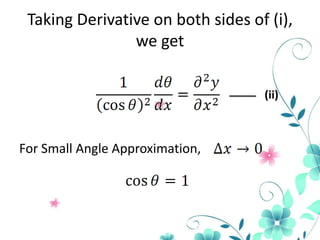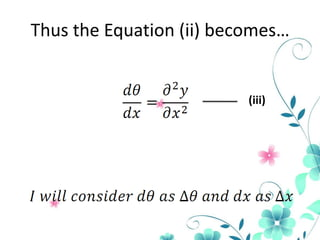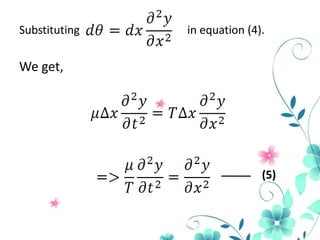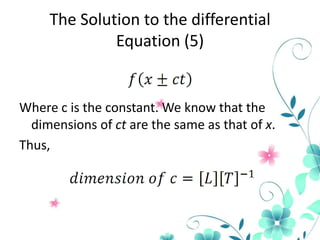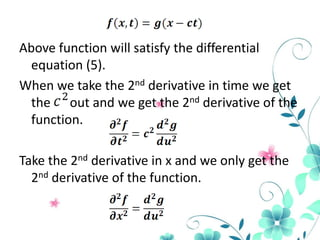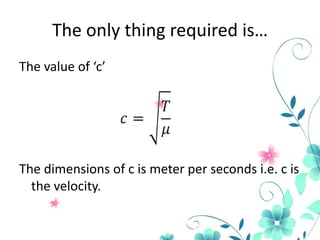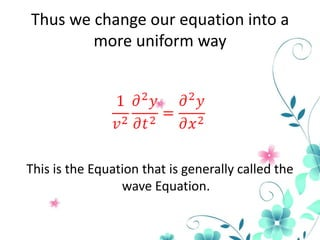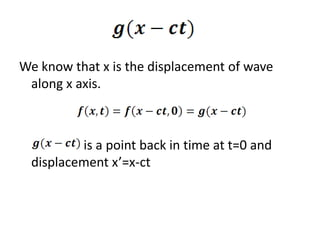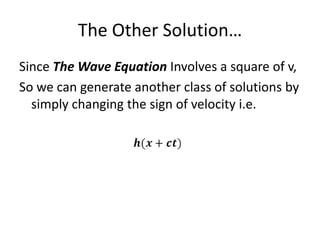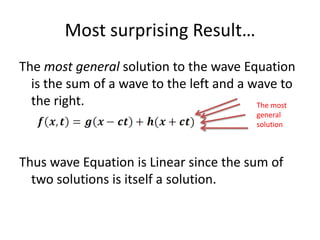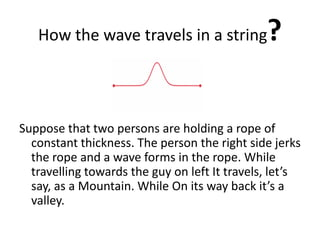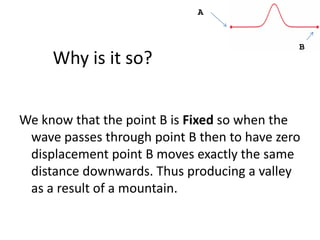1 de 23
Anúncio

### The wave eqution presentation

1. Applied Physics Presentation The Wave Equation Muhammad
2. What is the Wave Equation? The wave equation for a plane wave traveling in the x direction is: (A) where v is the phase velocity of the wave and y represents the variable which is changing as the wave passes.
3. Wave Equation in 2-Dimensions The mathematical description of a wave makes use of partial derivatives. In two dimensions the wave Equation takes the form (B)
4. The Wave in an Ideal String We start with a small portion of that rope. We have Tension ‘T’ and mass per unit length ‘µ’. If our displacement is not absurdly high then the Tension is the same on the both side.
5. Derivation of the Wave Equation We will only consider the motion in the Y direction. So That’s for small angles other wise all our assumptions will be wrong. When So now the Equation becomes: (1)
6. Appling Newton's Second Law The amount of mass that is in Since, here is From (1), (2)
7. What is ? We know that since the length of So, Where length. is is the amount of mass per unit .
8. So the Equation (2) becomes, (4)
9. Since in a limiting case we are going to make , We will find the tangent for θ, (i) We know that tangent of “theta” is always equal to the derivative in space (position). We used the partial derivative since we assume that its all happening on a instant in time.
10. Taking Derivative on both sides of (i), we get (ii) For Small Angle Approximation,
11. Thus the Equation (ii) becomes… (iii)
12. Substituting in equation (4). We get, (5)
13. The Solution to the differential Equation (5) Where c is the constant. We know that the dimensions of ct are the same as that of x. Thus,
14. Above function will satisfy the differential equation (5). When we take the 2nd derivative in time we get the out and we get the 2nd derivative of the function. Take the 2nd derivative in x and we only get the 2nd derivative of the function.
15. The only thing required is… The value of ‘c’ The dimensions of c is meter per seconds i.e. c is the velocity.
16. Thus we change our equation into a more uniform way This is the Equation that is generally called the wave Equation.
17. We know that x is the displacement of wave along x axis. is a point back in time at t=0 and displacement x’=x-ct
18. The Other Solution… Since The Wave Equation Involves a square of v, So we can generate another class of solutions by simply changing the sign of velocity i.e.
19. Most surprising Result… The most general solution to the wave Equation is the sum of a wave to the left and a wave to the right. The most general solution Thus wave Equation is Linear since the sum of two solutions is itself a solution.
20. ? How the wave travels in a string Suppose that two persons are holding a rope of constant thickness. The person the right side jerks the rope and a wave forms in the rope. While travelling towards the guy on left It travels, let’s say, as a Mountain. While On its way back it’s a valley.
21. A Why is it so? B We know that the point B is Fixed so when the wave passes through point B then to have zero displacement point B moves exactly the same distance downwards. Thus producing a valley as a result of a mountain.
Anúncio